# Different interpretations of the relationship between power

Keying Guan

Department of Mathematics, School of Science, Beijing Jiaotong University

Emailkeying.guan@gmail.com

Abstract: The relationship between the power P and the corresponding force F in the interaction of matter

P = F .v      (*)

is a basic physics formula. However, for more than a century, when using this formula to study the radiation pressure formed by light hitting the surface of a reflective object, the mainstream radiation pressure theory contradicts Einstein's radiation pressure theory on reflectors: the mainstream theory is considered to be The power absorbed by an object is equal to the sum of incident power and reflected power; Einstein believes that according to energy conservation, absorbed power is equal to the difference between incident power and reflected power. In addition to this basic contradiction, when using the formula (*) to calculate the radiation pressure of the illuminated object, the mainstream theory believes that the speed v in the formula is the speed of light c, while Einstein believes that it is the speed of the illuminated object measured by the observer. Clearly, these theories cannot be both correct. What is even more surprising is that this contradictory theory has not been discussed in the theoretical physics community since Einstein published the paper on such an important fundamental physics question, suggesting that there is still no clear consensus on what the formula actually means (*).

1. The contradiction between the mainstream light pressure theory

and Einstein's related theory

Since the April 2021 discussion on light sails and the Crookes radiometer, with the question, whether a more reflective surface or a less reflective surface can absorb more radiant energy and produce more light Pressure, the author has conducted a series of exploration and research on the theory of light pressure on ScienceNet. In particular, in 2022, the author discovered that related theories are related to the importance of the fundamental formula (*) in physics and mechanics. For relevant information, please refer to the following blog posts:

1).  没有不做功的力--马德堡半球实验、举重运动消耗的卡路里及辐射压公式 2022-07-01

(Note:This blog post is in Chinese. In order to make it easier for readers who do not understand Chinese to understand its main content, the author attaches its title and abstract after the references in this article.）

2).  Force is equivalent to Power 2022-07-02

4).  Rediscussing on the Force-Power equivalence 2022-07-18

During the exploration, the author first studied the Maxwell-Bartoli electromagnetic radiation (or light) pressure formula commonly used in mainstream physics circles,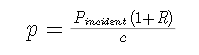(1)

Where p is the radiation pressure on the surface of the irradiated object, Pincident is the radiant energy per unit time perpendicular to the surface of the irradiated object (that is, the power directed to the unit area), c is the speed of light, R (0≦R≦1) is the reflection coefficient of the surface of the object. The theory of this formula was first proposed by the British physicist J.C.Maxwell in his masterpiece "A Treatise on Electricity and Magnetism" , Volume 2, Item 792 of the research topic <Energy and Stress of Radiation>, and Pointed out: "Hence in a medium in which waves are propagated there is a pressure in the direction normal to the waves, and numerically equal to the energy in unit of volume." Using the notation in this paper, his conclusion can be expressed as (original work not expressed in formulas)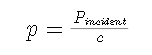(1*)

The right side of (1*) is "energy per unit volume". In item 793 <Sunlight>, Maxwell further estimated that the direct sunlight on the ground would cause a pressure of 0.4 milligrams per square meter based on the solar constant measured at that time.

Independent of Maxwell, in 1876, the Italian physicist Adolfo Bartoli proposed that the radiation pressure on the mirror surface is twice the pressure of (1*) according to his thinking on the second law of thermodynamics (refer to  ), that is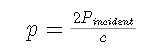(1**)

And also based on the solar constant at that time, he estimated that the sun directly hitting the ground would cause a pressure of 0.8 milligrams per square meter.

Synthesizing the ideas of Maxwell and Bartoli, in 1901, the Russian experimental physicist P Lebedew first gave the formula containing Specific form of the Maxwell-Bartoli formula for reflection coefficient (1).

The Austrian physicist Ludwig Eduard Boltzmann pointed out in his book <Ableitung des Stefan schen Gesetzes betreffend die Abhängigkeit der Wärmestrahlung von der Temperatur aus der electromagnetischen Lichttheorie> in 1884: the concept used by Maxwell, "Energy per unit volume", earlier (1856) by German chemist and physicist A. Krönig for the description of gas pressure in thermodynamics and statistical mechanics (reference  or English Wikipedia: https:// en.wikipedia.org/wiki/Kinetic_theory_of_gases).

In fact, the air pressure formula of an ideal gas deduced by Krönig is completely similar to (1**). The Pincident in the air pressure formula refers to the kinetic energy of the air flow perpendicular to the pressure surface to the unit area per unit time, that is, the power surface density of the air flow , the speed of light in the formula is changed to the speed of airflow.

A clearer theoretical explanation of the Maxwell-Bartoly formula today is in the English Wikipedia: https://en.wikipedia.org/wiki/Radiation_pressure

When Wikipedia introduced the formula (1), it replaced the incident power Picident in the formula (1*) with the Poynting vector S, and then used Pincident to represent the pressure p, and pointed out theoretically: The pressure formula is based on the The relationship between power and force (*), the speed v in the relationship is interpreted as the speed of light c, and the formula (1**) is recognized in the case of total reflection. Thus, in the case of partial reflection, mainstream theory actually admits that P in (*) is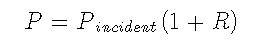(2)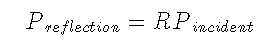(3)

Judging by the dimension, Preflection should also be a kind of power density, which is interpreted in this paper as the reflected power per unit area of the reflecting surface. Obviously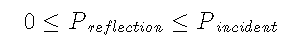In the same entry, when Wikipedia explains the radiation pressure (1**) or (1) of the reflective surface, it uses the following figure to describe more clearly why the mainstream theory uses the plus sign in the formula (1), or in the formula (1 **) using a multiple of 2,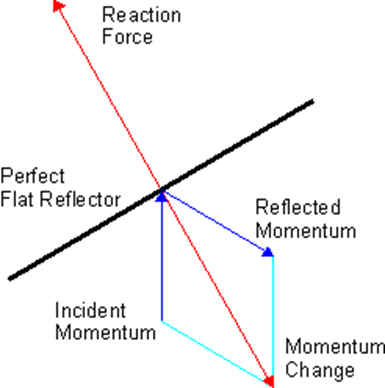Figure 1

This figure shows that the mainstream theory uses the conservation of momentum in elastic collisions to describe the pressure formed by electromagnetic radiation or airflow beams on the reflecting surface, but the relationship between power and force (*) is used in specific calculations. In fact, the combination of formula (*) and formula (2) just exposes that the mainstream theory violates the law of energy conservation.

According to the above facts, the author has pointed out in many papers published in the past two years that the formula (1) or (1**) violates the law of energy conservation, which makes it impossible to correctly explain the impact of the Crookes radiometer blades on the light. , and mistakenly regard the speed v in the formula (*) as the speed of light c, so that the light pressure calculated according to the formula (1) is too small.

Note: In order to prove that the light pressure is extremely small as calculated by Maxwell and Bartoli, there is a preconceived and suspicious Lebedev experiment. Because when Lebedev began to introduce his experiment in the paper , he first clearly explained his experimental motivation:

In seinem Lehrbuch 793 sagt Maxwell:

„Concentrirtes elektrisches Licht wird wahrscheinlich einen noch grösseren Druck ausüben (als die Sonnenstrahlung) und es ist nicht unmöglich, dass die Strahlen eines solchen Lichtes, wenn sie auf ein dünnes metallisches Blättchen, das in einem Vacuum fein aufgehängt ist, fallen, an diesem einen be In obachtbaren mechanischen Effect ausüben.

Als ich meine Versuche beginnen wollte, schien mir die von Maxwell vorgeschlagene Versuchsanordnung aussichtslos, weil bereits Zöllner   diesen Weg ohne Erfolg eingeschlagen und auch „darauf aufmerksam gemacht hat, dass der von Maxwell theoretisch erlangte Wert (des Lichtdruckes) ungefåhr 100000 mal kleiner sei als der von Crookes in einem speciellen Falle beobachtete Wert der Resultate",   Wenn man auch darauf rechnen konnte, diese störenden radiometrischen Wirkungen ganz erheblich zu verkleinern, so glaubte ich denr.och, dass nur eine solche Einrichtung zum Ziele führen könnte, in welcher diese radiometrischen Kräfte compensirt sind.

The English translation is:

In his textbook 793 Maxwell says:

"Concentrated electric light will probably exert a still greater pressure (than solar radiation), and it is not impossible that the rays of such light, when they fall on a thin metallic plate suspended in a vacuum, on this one be In exert an observable mechanical effect.”

When I wanted to start my experiments, the experimental arrangement proposed by Maxwell seemed hopeless to me, because Zöllner  had already taken this path without success and also "pointed out that the value (of the light pressure) theoretically obtained by Maxwell is about 100,000 times smaller than the value of the results observed by Crookes in a special case".  Even if one could count on considerably reducing these disturbing radiometric effects, I nevertheless believed that only such a device could lead to the goal in which these radiometric forces are compensated.

It can be seen from the paper  that in order to achieve the expected experimental value, Lebedev filtered out infrared light, ultraviolet light, etc. in the experiment; only the measurement of thermal power represents the measurement of incident light power, especially without measuring the reflected light Power; only one piece of the pressed surface is measured at a time, instead of simultaneously checking the response of the reflective surface and the non-reflective surface to the same radiation as with the Crookes radiometer, and so on. In fact, these are not conducive to the accurate measurement of the actual light pressure. In order to save space, the author will introduce the problems involved in this experiment in detail in another article.

In 2022, the author further noticed that in fact, as early as 1905, German-born physicist A. Einstein wrote in his famous first work on special relativity "Zur Elektrodynamik bewegter Körper"  (“ On the electrodynamics of moving bodies"), when discussing the radiation pressure formed by the light energy acting on the mirror, he explicitly used the law of conservation of energy to explain the cause of the radiation pressure:In <ON THE ELECTRODYNAMICS OF MOVING BODIES> , the corresponding English content (in page 18-19) is

The energy (measured in the stationary system) which is incident upon unit area of the mirror in unit time is evidently A2(c cos φ−v)/8π. The energy leaving the unit of surface of the mirror in the unit of time is A'''2(−c cos φ''' + v)/8π. The difference of these two expressions is, by the principle of energy, the work done by the pressure of light in the unit of time. If we set down this work as equal to the product Pv, where P is the pressure of light, we obtain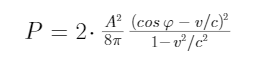(4)

In agreement with experiment and with other theories, we obtain to a first approximation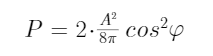(5)

In the quotation, Einstein, using the customary usage in the physics circle at that time, used P to represent the pressure p, and V to represent the speed of light.

The pressure formula in Einstein's original work has no serial numbers, (4) and (5), and this paper adds these two serial numbers for the convenience of description.

Apparently, the "other theories" mentioned in Einstein's paper refer to the light pressure formula (1) of the mainstream theory. It can be seen that Einstein's theory is fundamentally different from "other theories" in the following three points:

i) The basis of Einstein's light pressure theory is based on the principle of energy conservation. It is considered that the work done by the electromagnetic radiation per unit area of the mirror per unit time (that is, the power absorbed by the unit area mirror, denoted as Pabsorbed) is equal to the energy per unit area of the radiation incident on the mirror per unit time (measured by the observer in the static system) ( That is, the difference between the incident power Pincident) and the energy per unit area leaving the mirror per unit time (ie the reflected power Preflection),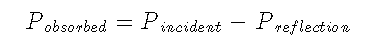(6)

This is undoubtedly in favor of the author.

According to (2) and (3), it can be seen that the mainstream theory holds that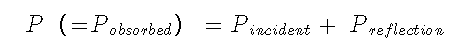（7）

ii) Einstein's light pressure theory was just when he created the basis of special relativity, in order to                 fully use the effect of Lorentz transformation, his model assumes a static system K and a moving                     system moving with the mirror k, so there is automatically a velocity v of the moving system k                           relative to the stationary system.

But the era when the formula (1) is proposed has not yet established the theory of relativity, and this                 speed does not exist in the formula.

iii) Einstein also stated from the mechanics formula (*) that,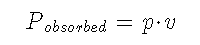(8)

The mainstream theory holds that,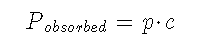(9)

What is surprising is that according to Einstein's above theory, he should have derived a radiation pressure formula that is completely different from the mainstream theory, such as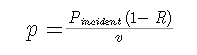(10)

Although Einstein knew that his theory was different from other theories, the formulas he gave were (4) and (5). And just as he judged, what he got turned out to be "as far as the first approximation is concerned, we get a result that is consistent with the experiment (obviously referring to Lebedew's light pressure experiment ), and also consistent with other theories".

The author did a test: due to Einstein's paper, the factors A2/(8π) and A'''2/(8π) in the formula represent the energy in the unit volume of the incident and reflected electromagnetic fields respectively, so the formula (5) It is indeed consistent with the formula (1**)!

What exactly does this contradictory result reflect? Faced with this serious and interesting question, the author found that Einstein's argument had the following misjudgments:

One is that, without any derivation, he gives a judgment directly:

The energy (measured in the stationary system) incident on the mirror per unit area per unit time is obviously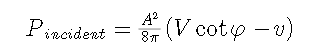(11)

The energy per unit area leaving the mirror per unit time is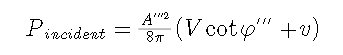(12)

Although his thesis has demonstrated in detail that the factors A2/(8π) or A'''2/(8π) in the above two formulas meet the requirements of the Lorentz transformation in special relativity, but the speed factor (cosφ -v) and (cosφ’’’ v) does not demonstrate whether it meets the requirements of the Lorentz transformation. In fact, these two factors are invariant only under the Galileo transformation of Newtonian mechanics. When the speed v is in the same or opposite direction to the speed of light V, according to the derivation of this paper. It can be found that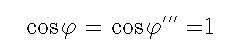or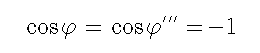If the velocity v is not equal to zero, one of the above two velocity factors must become the superluminal speed, and the superluminal speed cannot exist in the framework of the special theory of relativity, and cannot be allowed. There is no doubt that these two expressions show an oversight in Einstein's judgment.

In addition, the formula (5) deduced by Einstein also obviously contradicts his ideas on the generation of light pressure:

When accurately calculated according to the deliberated formula he gave, if the relative velocity v is equal to zero, there must be φ = 0, φ’’’=0, and in this case Pabsorbed must be equal to zero. According to Einstein's thinking, it can be directly judged that the power absorbed by the mirror must be zero, which means that the mirror will not be subject to any pressure.

However, when using formulas (11) and (12) without rigorous scrutiny, in the case that the speed v is not equal to 0, formula (4) can be deduced first, and then v = 0, thus obtaining the formula ( 5). (5) means that the mirror will still be subjected to (non-zero) light pressure.

This contradiction further shows that the formulas (11) and (12) given by Einstein without proof must have errors.

Another judgment error in Einstein's paper is manifested in the understanding of the velocity v in formula (*) or (8).The velocity v defined in advance in his paper has nothing to do with the existence of optical radiation pressure. But this is by no means the speed that the formula (*) refers to.

2. The speed in the equivalence relationship between power and force

The discussion in the previous section fully shows the contradiction between Einstein’s light pressure theory and the mainstream theory, and also shows that when using the formula (*) to calculate the light pressure, the two theories have different understandings of the speed in the formula.

In several published blog posts mentioned at the beginning of this article, the authors have shown that when the formula (*) is expressed in the International System of Units (SI), where the velocity v of must be the unit velocity, ie one meter (m) per second (s). In this paper, unit velocity is denoted as vunit,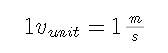(13)

In fact, the reason is very simple:

According to the definition of power P, it is the rate of change of energy E(t) in matter interaction with time, that is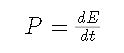(14)

According to the International System of Units, the unit of power is Watt (Watt, abbreviated as W), and its value is equal to one energy unit per second, that is, Joule, abbreviated as J, so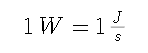(15)

Since a unit of energy J is equal to 1 Newton-meter (Newton-Meter), and N (Newton) is the standard unit of force, then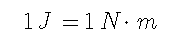(16)

therefore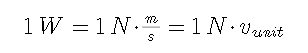(17)

Since W is the unit of power P and N is the unit of force F, the relationship (*) should be expressed exactly as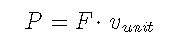(18)

The different interpretations of velocity v in formula (*) by mainstream theory and Einstein’s theory further illustrate the necessity of converting formula (*) into formula (18), and also show that both Einstein’s and mainstream theory’s explanations are wrong .

From the derivation process of (*) to (18), it can be seen that the velocity v in the relationship between power and force (*) is not related to the instantaneous velocity when matter interacts. In fact, for the same intensity of instantaneous force F, the object under force will show different speeds under different circumstances.

A common example: a car with a fixed load and a fixed output power (and therefore a fixed output thrust) must drive at different speeds when driving on roads with different frictional resistances.

Study another example worth thinking about:

Assuming that a helicopter has hovered in the air, according to engineering needs, use the helicopter's wire rope to lift and maintain a 100 kg object to a certain height. In addition to the power a helicopter needs to maintain a hover without dangling heavy loads, the helicopter also needs to provide enough power to lift heavy loads. According to equation (18), this added lifting force must be slightly greater than 100 kg (by weight) or 980 Newtons.

Especially when the raised object is kept at the required height, the pilot also needs to let the helicopter provide an additional 980 watts of power to support the weight of 100 kg, otherwise the raised object cannot maintain that stable height, although in this case , this gravitational force is a static force. This fact shows that the static force must also do work according to the formula (18).

3. The relationship between power and force is an important equivalence relationship

Einstein's special theory of relativity reveals the equivalence relationship between energy E and mass m of matter,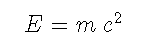(19)

The significance and importance of the equivalence relation (19) need not be repeated here.

Since the refined relationship between power and force (18) is completely similar to the equivalent relationship (19), and the factors c2 and vunit in the formula are universal constants required by the balance dimension, the equation (18 ) is actually an equivalent relationship between power and force.

Obviously, the physics community has ignored the contradiction between Einstein and the mainstream theory of light pressure explained in this paper, let alone the recognition of the equivalence relationship between power and force.

Facing the equivalence relationship between power and force is not only meaningful for the correct understanding of light pressure, but also can correct the misunderstanding of classical mechanics that static force does not do work, so that more natural phenomena can be discovered and explained, and the research field of physics more extensive.

The author will explore some related issues in another article.

References

 J. C. Maxwell, A Treatise on Electricity and Magnetism (1St ed.), Vol. 2, Oxford, 1873.

 A. Bartoli, Ezner's Bep. d. Phys. 21. p. 198. 1884, übersetzt aus Nuovo Cimento 16. p. 195. 1883.

 P. Lebedew,  Untersuchen uber die druckkrafte des Lichtes, Annalen der Physik 46, 432 (1901).

 Ludwig Eduard Boltzmann ,  Ableitung des Stefan schen Gesetzes betreffend die Abhängigkeit der Wärmestrahlung von der Temperatur aus der electromagnetischen Lichttheorie, pp. 291-294 in: Annalen der Physik und Chemie, Band 22, Heft 2, No. 6, 1884

 Krönig, A. (1856), Grundzüge einer Theorie der Gas, Annalen der Physik 99 (10): 315–322

 Einstein, Albert (1905d) [Manuscript received: 30 June 1905]. Written at Berne, Switzerland. "Zur Elektrodynamik bewegter Körper". Annalen der Physik (Submitted manuscript) (in German). Hoboken, New Jersey (published 10 March 2006). 322 (10): 891–921.

Appendix: English title and abstract of the blog post

There is no force that does not do work

-- Magdeburg hemispheric experiment, calories burned in weightlifting and radiation pressure formula

Abstract: This paper points out that the combination of the law of conservation of energy and the law of conservation of momentum implies an important law that is often ignored because it is not obvious when it comes to "static force" problems, that is, there is no force that does not do work. This law can be precisely expressed as force is equivalent to power, that is, every newton of force acting on material is equivalent to outputting 1 watt of power. This law is reflected not only in the Magdeburg hemisphere experiment, but also in the calories consumed by weightlifters, and also in the plastic deformation, heat generation, fatigue, and fracture degree of the extruded (or streched) material per unit time. Ignoring this rule will result in misjudgment of practical problems. For example, many people mistakenly believe that a person who is standing and carrying a weight is not doing work when he is not pushing the weight higher further, etc., so he consumes the same amount of physical energy as if he were not carrying the weight. Such misjudgments are more seriously reflected in the Maxwell-Bartoli radiation pressure formula (including Krönig's pressure formula for ideal gas) with reflection coefficients in modern physical science theories. This formula erroneously predicts that the radiation pressure on the surface of the strongly reflective material with a small ability to receive radiation is greater than the radiation pressure on the surface of the weakly reflective material with a large ability to receive radiation. This prediction both contradicts the experimental facts of the Crookes radiometer and grossly underestimates Crookes' scientific contributions. Using the above rules, this paper not only gives the mechanical estimation of the calories consumed by weightlifters in a weightlifting activity, but also further, comprehensively uses the methods of thermodynamics and statistical physics and the precise solution of the elastic collision of two particles, gives the electromagnetic radiation beam The formula for light pressure and ideal airflow pressure,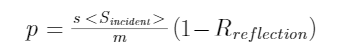where <Sincidentis the power of the incident flow through a unit area, Rreflection is the reflection coefficient of the illuminated object, s is the unit time (1 second), and m is the unit length (1 meter).

https://blog.sciencenet.cn/blog-553379-1382875.html

## 全部精选博文导读

GMT+8, 2023-10-4 00:38